Test: Buoyancy & Floatation - 2

# Test: Buoyancy & Floatation - 2 - Mechanical Engineering

Test Description

## 10 Questions MCQ Test Fluid Mechanics for Mechanical Engineering - Test: Buoyancy & Floatation - 2

Test: Buoyancy & Floatation - 2 for Mechanical Engineering 2023 is part of Fluid Mechanics for Mechanical Engineering preparation. The Test: Buoyancy & Floatation - 2 questions and answers have been prepared according to the Mechanical Engineering exam syllabus.The Test: Buoyancy & Floatation - 2 MCQs are made for Mechanical Engineering 2023 Exam. Find important definitions, questions, notes, meanings, examples, exercises, MCQs and online tests for Test: Buoyancy & Floatation - 2 below.
Solutions of Test: Buoyancy & Floatation - 2 questions in English are available as part of our Fluid Mechanics for Mechanical Engineering for Mechanical Engineering & Test: Buoyancy & Floatation - 2 solutions in Hindi for Fluid Mechanics for Mechanical Engineering course. Download more important topics, notes, lectures and mock test series for Mechanical Engineering Exam by signing up for free. Attempt Test: Buoyancy & Floatation - 2 | 10 questions in 30 minutes | Mock test for Mechanical Engineering preparation | Free important questions MCQ to study Fluid Mechanics for Mechanical Engineering for Mechanical Engineering Exam | Download free PDF with solutions
 1 Crore+ students have signed up on EduRev. Have you?
Test: Buoyancy & Floatation - 2 - Question 1

### When a body, floating in a liquid is given a small angular displacement, it starts oscillating about a point known as

Detailed Solution for Test: Buoyancy & Floatation - 2 - Question 1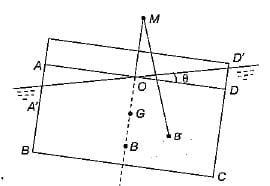Where M is metacentre and θ is small angle of hill through which a body is tilted.
Hence metacenter is a point about which a body oscillate when tilted or given a small angular displacement.

Test: Buoyancy & Floatation - 2 - Question 2

### The time of oscillation of a floating body is given by Where K = Radius of Gyration of the floating body about its centre of gravity h = Metacentric height of the floating body

Detailed Solution for Test: Buoyancy & Floatation - 2 - Question 2

Time of oscillation of a floating body is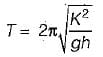h = metracentric height

Test: Buoyancy & Floatation - 2 - Question 3

### The time of oscillation of a floating body with increase in metacentric height will be

Detailed Solution for Test: Buoyancy & Floatation - 2 - Question 3

Time of oscillation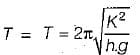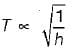as h increases, time of oscillation decreases

Test: Buoyancy & Floatation - 2 - Question 4

A floating body is in stable equilibrium when

Detailed Solution for Test: Buoyancy & Floatation - 2 - Question 4

For stable equilibrium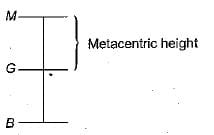Test: Buoyancy & Floatation - 2 - Question 5

A submerged body will be in stable equillibrium. if

Detailed Solution for Test: Buoyancy & Floatation - 2 - Question 5

For stable equilibrium of submerged body: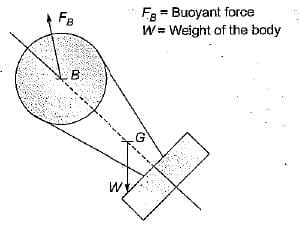Hence for stable equilibrium of submerged body centre of buoyancy B should be above G.

Test: Buoyancy & Floatation - 2 - Question 6

Which one of the following is the conditions for stable equilibrium for a floating body

Detailed Solution for Test: Buoyancy & Floatation - 2 - Question 6

For stable equilibrium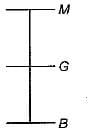For stable equilibrium metacenter is above the center of gravity.

Test: Buoyancy & Floatation - 2 - Question 7

How is the metacentric height (GM) expressed
Where I = Moment of inertia of the plan of the floating body at the water surface
V = Volume of the body submerged in water
BG = Distance between the center of gravity (G) and the center of Buoyancy (B)

Detailed Solution for Test: Buoyancy & Floatation - 2 - Question 7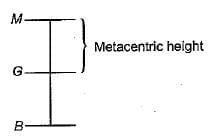Metacentric height,
GM = BM - BG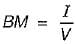Where I = Area moment of inertia of top view about longitudinal axis
V = Volume of the fluid displaced
Hence,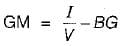Test: Buoyancy & Floatation - 2 - Question 8

An odd shaped body weighing 7.5 kg and occupying 0.01 m3 volume wiil be completely submerged in a fluid having specific gravity of

Test: Buoyancy & Floatation - 2 - Question 9

A cylindrical body of cross-sectional area A height H and density ρs is immersed to a depth h in a liquid of density ρ and tied down to bottom with a string then the tension in the string is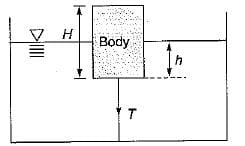Detailed Solution for Test: Buoyancy & Floatation - 2 - Question 9

w + T =  FB = weight of fluid displaced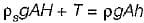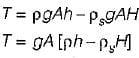Test: Buoyancy & Floatation - 2 - Question 10

A float valve of the ‘ball-cock’ type required to close an opening of a supply pipe feeding a cistern as shown in the given figure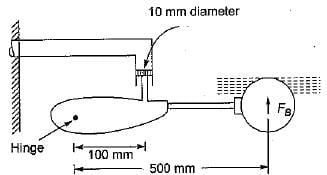The buoyant force FB required to be exerted by the float to keep the valve closed against a pressure of 0.28 N/mm2 is

Detailed Solution for Test: Buoyancy & Floatation - 2 - Question 10

Taking moment about hinge We get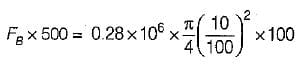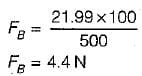## Fluid Mechanics for Mechanical Engineering

50 videos|90 docs|76 tests
Information about Test: Buoyancy & Floatation - 2 Page
In this test you can find the Exam questions for Test: Buoyancy & Floatation - 2 solved & explained in the simplest way possible. Besides giving Questions and answers for Test: Buoyancy & Floatation - 2, EduRev gives you an ample number of Online tests for practice

## Fluid Mechanics for Mechanical Engineering

50 videos|90 docs|76 tests(Scan QR code)